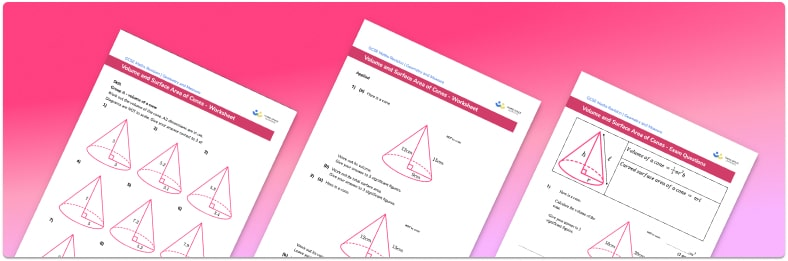# Volume And Surface Area Of A Cone WorksheetHelp your students prepare for their Maths GCSE with this free volume and surface area of cones worksheet of 34 questions and answers

• Section 1 of the volume and surface area of cones worksheet contains 27 skills-based volume and surface area of cones questions, in 3 groups to support differentiation
• Section 2 contains 4 applied volume and surface area of cones questions with a mix of worded problems and deeper problem solving questions
• Section 3 contains 3 foundation and higher level GCSE exam style volume and surface area of cones questions
• Answers and a mark scheme for all volume and surface area of cones questions are provided
• Questions follow variation theory with plenty of opportunities for students to work independently at their own level
• All questions created by fully qualified expert secondary maths teachers
• Suitable for GCSE maths revision for AQA, OCR and Edexcel exam boards

• This field is for validation purposes and should be left unchanged.

You can unsubscribe at any time (each email we send will contain an easy way to unsubscribe). To find out more about how we use your data, see our privacy policy.

### Volume and surface area of cones at a glance

A cone is a 3d shape. It has a circular base and one continuous face which tapers to a point, often referred to as the apex. The apex of a right circular cone is directly above the centre of the circular base.

To find the volume of a cone we use the formula: V = one third✖πr2✖h where r is the radius of the base of the cone, and h is the perpendicular height of the cone.

For example, the volume of the cone with radius r=3cm and height h=10cm is V=30π.

To find the total surface area of a cone, we find the area of each face and add them together. The area of the base of the cone is found using the formula: A = π✖r2 . The curved surface area of a cone is found using the formula: A = πrl, where r is the radius of the base and l is the slant height of the cone. These areas are then added to give the total surface area of the cone. Note, if you flatten the curved surface area of the cone, you will get a major sector of a circle with radius l (the slant height of the cone).

If we do not know the slant height l of the cone, we can use Pythagoras’ Theorem where the base of the right-angled triangle is the radius of the base of the cone, and the perpendicular height of the right-angled triangle is the height of the cone.

Looking forward, students can then progress to additional 3D shapes worksheets and more geometry worksheets, for example an angles in polygons worksheet or how to calculate volume worksheet.For more teaching and learning support on Geometry our GCSE maths lessons provide step by step support for all GCSE maths concepts.

## Do you have KS4 students who need more focused attention to succeed at GCSE?There will be students in your class who require individual attention to help them succeed in their maths GCSEs. In a class of 30, it’s not always easy to provide.

Help your students feel confident with exam-style questions and the strategies they’ll need to answer them correctly with our dedicated GCSE maths revision programme.

Lessons are selected to provide support where each student needs it most, and specially-trained GCSE maths tutors adapt the pitch and pace of each lesson. This ensures a personalised revision programme that raises grades and boosts confidence.

Find out more